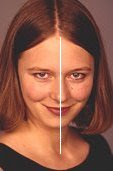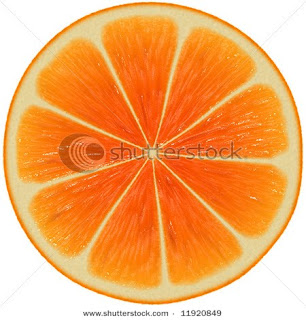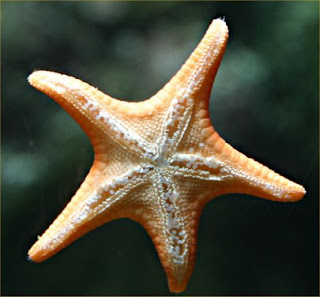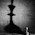## Search This Blog

THE BEAUTY OF MATHEMATICS- Collected from The Internet and Various Books to enrich The students and Teachers. SUPPORT with YOUR COMMENTS ...

## Monday, August 30, 2010

### MATHS AND NATURE

"The laws of nature are but the mathematical thoughts of God"
- Euclid

Mathematics is everywhere in this universe. We seldom note it. We enjoy nature and are not interested in going deep about what mathematical idea is in it. Here are a very few properties of mathematics that are depicted  in nature.
SYMMETRY

Symmetry is everywhere you look in nature .

Symmetry is when a figure has two sides that are mirror images of one another. It would then be possible to draw a line through a picture of the object and along either side the image would look exactly the same. This line would be called a line of symmetry.

There are two kinds of symmetry.

One is bilateral symmetry in which an object has two sides that are mirror images of each other.

The human body would be an excellent example of a living being that has bilateral symmetry.Few more pictures in nature showing bilateral symmetry.

The other kind of symmetry is radial symmetry. This is where there is a center point and numerous lines of symmetry could be drawn.

The most obvious geometric example would be a circle.Few more pictures in nature showing radial symmetry.SHAPES

Geometry is the branch of mathematics  that describes shapes.

Sphere:

A sphere  is a perfectly round geometrical object in three-dimensional space, such as the shape of a round ball.

The shape of the Earth is very close to that of an oblate spheroid, a sphere flattened along the axis from pole to pole such that there is a bulge around the equator.

Hexagons:

Hexagons are six-sided polygons, closed, 2-dimensional, many-sided figures with straight edges.

For a beehive, close packing is important to maximise the use of space. Hexagons fit most closely together without any gaps; so hexagonal wax cells are what bees create to store their eggs and larvae.

Cones:

A cone is a three-dimensional geometric shape that tapers smoothly from a flat, usually circular base to a point called the apex or vertex.

Volcanoes form cones, the steepness and height of which depends on the runniness (viscosity) of the lava. Fast, runny lava forms flatter cones; thick, viscous lava forms steep-sided cones.

Few more cones in nature:

Parallel lines:

In mathematics, parallel lines stretch to infinity, neither converging nor diverging.

These parallel dunes in the Australian desert aren't perfect - the physical world rarely is.

Fibonacci spiral:

If you construct a series of squares with lengths equal to the Fibonacci numbers (1,1,2,3,5, etc) and trace a line through the diagonals of each square, it forms a Fibonacci spiral.

Many examples of the Fibonacci spiral can be seen in nature, including in the chambers of a nautilus shell.

1.fascinating (Y)

2.just awesome!!helped ma project a lot.. thankyou

1.helped my project to thx alot!

2.I have a project too for GT

3.3.awesome

4.great work ..

5.6.Well described!!! Thanx!

7.two best friends are maths nd nature!!!!!!!!!

8.too..good!!!!!!!!

9.Thanks for Sharing!!!

10.amazing..

11.Awesome! It would have been even more awe -inspiring if PHI had been mentioned.

12.THANNKS!!

13.Excellent. Thank you for sharing.

14.15.Very helpful thank you so much

16.17.thank you soooooo much
helped alot with project

18.Its good but can also tell me more by giving more details on this topics.....I hav to make a project...plz............

1.what else do u want???? it is just AWESOME!!!!!!

2.Hehehe seriously.. What else do u want? its srsly AWESOME ! :p

19.thanks u...It help me to make a project...

20.21.22.23.thanku very much helped me in my project

24.very useful

25.thanx they helped me alot

26.excellent and very useful!

27.thnkuuuuu alot .. !! :) :)

28.fantastic content

29.helped very much in my project...ty!!!!!
very useful...!!!!!

30.really useful.

31.thamks a lot...was useful for my project

32.its just awesome !a big help in my project

33.thank you to help me in my school project

34.thnqqq very much !!!

35.36.VERY VERY USEFUL.. THANKS A LOT !

37.Thank you all for your support!!

38.It's of lot help....

39.thanks !!!!!!!!!! its the only thig i found about beauti of maths on net which was worth my project

40.thank u veryyyyyyyyyyy much helped me in my project

41.The Beauty of Mathematics and the Love of God! This is TOO cool!
Just the math part is good enough, the end is even better.
Beauty of Mathematics!!!!!!!

1 x 8 + 1 = 9
12 x 8 + 2 = 98
123 x 8 + 3 = 987
1234 x 8 + 4 = 9876
12345 x 8 + 5 = 98765
123456 x 8 + 6 = 987654
1234567 x 8 + 7 = 9876543
12345678 x 8 + 8 = 98765432
123456789 x 8 + 9 = 987654321

1 x 9 + 2 = 11
12 x 9 + 3 = 111
123 x 9 + 4 = 1111
1234 x 9 + 5 = 11111
12345 x 9 + 6 = 111111
123456 x 9 + 7 = 1111111
1234567 x 9 + 8 = 11111111
12345678 x 9 + 9 = 111111111
123456789 x 9 +10= 1111111111

9 x 9 + 7 = 88
98 x 9 + 6 = 888
987 x 9 + 5 = 8888
9876 x 9 + 4 = 88888
98765 x 9 + 3 = 888888
987654 x 9 + 2 = 8888888
9876543 x 9 + 1 = 88888888
98765432 x 9 + 0 = 888888888

Brilliant, isn't it?

And look at this symmetry:

1 x 1 = 1
11 x 11 = 121
111 x 111 = 12321
1111 x 1111 = 1234321
11111 x 11111 = 123454321
111111 x 111111 = 12345654321
1111111 x 1111111 = 1234567654321
11111111 x 11111111 = 123456787654321
111111111 x 111111111 = 12345678987654321

Mind Boggling...

Now, take a look at this...

101%
From a strictly mathematical viewpoint:

What Equals 100%?

What does it mean to give MORE than 100%?

Ever wonder about those people who say they
are giving more than 100%?

We have all been in situations where someone wants you to

GIVE OVER 100%...

What equals 100% in life?

Here's a little mathematical formula that might help

If:
A B C D E F G H I J K L M N O P Q R S T U V W X Y Z

Is represented as:
1 2 3 4 5 6 7 8 9 10 11 12 13 14 15 16 17 18 19 20 21 22 23 24 25 26.

Then:
H-A-R-D-W-O-R-K

8+1+18+4+23+15+18+11 = 98%

And:

K-N-O-W-L-E-D-G-E

11+14+15+23+12+5+4+7+5 = 96%

But:
A-T-T-I-T-U-D-E
1+20+20+9+20+21+4+5 = 100%

THEN, look how far the love of God will take you:

L-O-V-E-O-F-G-O-D

12+15+22+5+15+ 6+7+15+4 = 101%

Therefore, one can conclude with mathematical certainty that:

While Hard Work and Knowledge will get you close, and Attitude will
get you there, It's the Love of God that will put you over the top!

Have a nice day!

1.Thanks for sharing How about ACHIEVING 101%?

2.amazing facts both by u and this website

42.Thanks to all for your support

43.you are jus amazing!! this is exctly wt i neeeded fr my maths assignmnt

44.I HATE MATH....
But this site made me love it for once...:D
THANKS A TON!!!

45.I Liked it...
nice site!

46.I like it...
Enjoyed A LOt n helped me a lots in my assingement !
three cheers fo d sit
(y)

47.48.This will surely help me in my project.Found the right site.Thnx a lot! :)

49.Wow! My Maths project done!

50.Great work...helped in my project alot.....thx

51.thank u very much u helped me to do my project

52.fabulous

53.just awesome

54.thnx it helped me a lot

55.wow this is too cool
got to know very much thru this

56.thnx it helped for my maths activity

57.thnxx it helped me

58.THANK YOU - FOR THIS INFORMATION !!
GOOD WORK DONE !

59.60.super..............

61.superrrrrrrrrrrr

62.Iam soo happi vth u...thanx..a lot..4 ur hlp...

63.thanks helped me in my project

64.It helped me a lot....thanx...:)

65.it's really helpful for my holiday homework
thanks a lot

66.It helped me with a project

67.super it helped me alout tanx..

68.I hate maths but this kind of maths is really good. thx a lot....................(:

69.great and interesting proofs about the symmetry between nature and math

70.helped my in my project to thx a lot!

71.72.i really wanted this kind of info..

73.cool....(:

74.